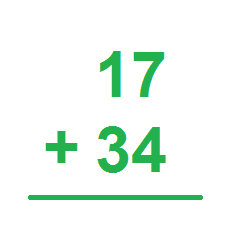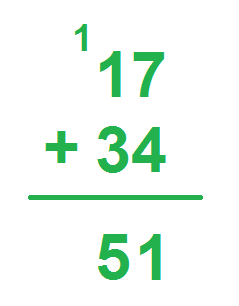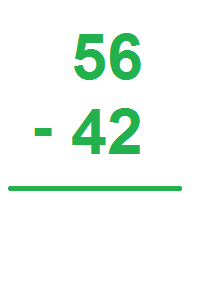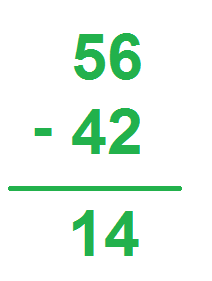# Algorithm Lesson for Kids

Instructor: Audrey Akins

Audrey has more than a decade of experience teaching elementary. She has a bachelor's in journalism and a master's in education.

When you began to add and subtract, you may have learned using objects you can hold, like blocks. In this lesson, you'll learn what an algorithm is you can move from using objects to using an algorithm.

## What is an Algorithm?

An algorithm is a step-by-step way of solving math problems faster than counting one-by-one. It's a set of instructions you use to find the answer faster, much like following directions to the store or following the instructions in a recipe for cookies. In this lesson, we'll look at the algorithms for addition, subtraction and multiplication.

The standard algorithm for addition has two basic steps:

1. Arrange the numbers vertically (on top of each other) so the place values line up (the ones places are in the same column, the tens places are in the same column, and so forth).
2. Starting from the right, add the digits in each place value. Carry over sums larger than 10 as you go, if necessary.

### Example

Say you have 17 oatmeal cookies and 34 cupcakes. You want to know how many total snacks you have. Let's work through our algorithm:

1. Arrange the numbers vertically so that the place values line up:2. Starting from the right, add the digits in each place value. Carry over sums that are 10 or larger as you go, if necessary:Notice that, since 7 + 4 is 11 (greater than 10), we carried over the 1 into the tens place. Then, we added the tens places (including our carried over number) to find out answer: 51.

## Subtraction Algorithm

The standard algorithm for subtraction is similar to the algorithm for addition. Let's look at each step:

1. Arrange the numbers vertically so the place values line up. Make sure the number you are subtracting from is on top, and the number being subtracted is on the bottom.
2. Starting from the right, subtract the top digit from the bottom in each place value. Borrow from the larger place value when necessary.

### Example

Your best friend gives you 56 Skittles and tells you that 42 of them are green and the rest are orange. To find the number of orange skittles, you can solve for 56 - 42. Let's use our algorithm:

1. Arrange the numbers vertically, with the number being subtracted from on top:2. Starting from the right, subtract the top digit from the bottom in each place value. Borrow from the larger place value when necessary:\

You'll notice that, in this example, we didn't need to borrow from the larger place value. If the top digit were smaller than the bottom digit, you would need to borrow from the top digit in the left column.

## Multiplication Algorithm

Things get a bit more complicated with the multiplication algorithm, and the steps can change depending on how many digits are being multiplied. Let's look the steps for multiplying a two-digit number by a one-digit number:

To unlock this lesson you must be a Study.com Member.

### Register to view this lesson

Are you a student or a teacher?

#### See for yourself why 30 million people use Study.com

##### Become a Study.com member and start learning now.
Back
What teachers are saying about Study.com

### Earning College Credit

Did you know… We have over 200 college courses that prepare you to earn credit by exam that is accepted by over 1,500 colleges and universities. You can test out of the first two years of college and save thousands off your degree. Anyone can earn credit-by-exam regardless of age or education level.